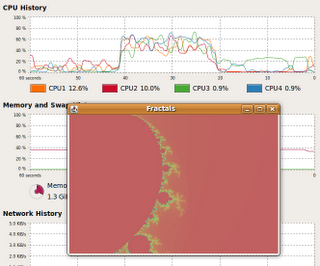## Tuesday, 3 February 2009

### Speeding up the Mandlebrot app

Previously I had some simple code for calculating the Mandelbrot fractal. Unfortunately, it was dog slow.

The primary reason is the use of `BufferedImage`. This is fine when you're loading an image from a file, but it's a big useless to go through a sequence setting each and every pixel with `setRGB`.

When you've already got all the data in memory, `MemoryImageSource` is a much better bet. You can create one with a 1D array of pixel data (with a specified width/height). `MemoryImageSource` implements the `ImageProducer` interface which means that any AWT derived component can use `createImage` to make an `Image`.

Firstly, I converted over the (x,y) set to just a list of numbers where (given the height and width) you can work out the corresponding pixel index, then I can just apply `pmap` (see also Ray Tracing)to calculate all the pixels in parallel.

`(defn calculate-pixels []  (let [pixels (range 0 (* *width* *height*))]    (pmap (fn [p]             (let [row (rem p *width*) col (int (/ p *height*))]              (get-color (process-pixel (/ row (double *width*)) (/ col (double *height*))))))          `

Next we need the interop to go from my sequence of pixel values to an integer array.

`(defn simple-mandlebrot [w h]  (let [x (int-array (calculate-pixels))]    `

`int-array` converts between a list of integers to a plain old Java array. I could probably use a Java Array for the whole thing, and not use seq, but I doubt this is an appreciable performance difference.

These changes have made a huge difference, instead of taking minutes to render a 512x512 image it takes a few seconds and all my CPU cores are occupied.